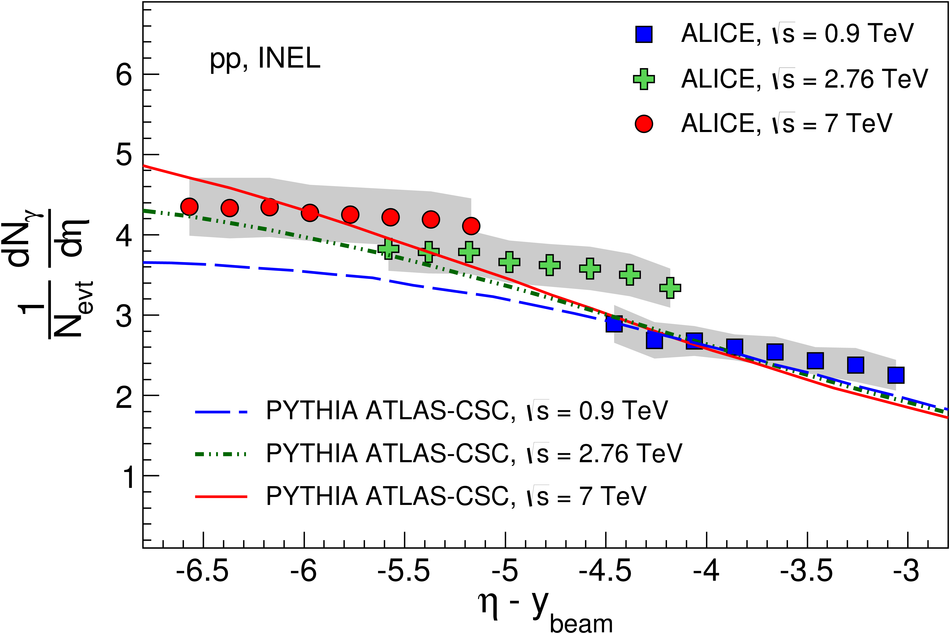# Inclusive photon production at forward rapidities in proton-proton collisions at $\sqrt{s}$ = 0.9, 2.76 and 7 TeV

The multiplicity and pseudorapidity distributions of inclusive photons have been measured at forward rapidities ($2.3 < \eta < 3.9$) in proton-proton collisions at three center-of-mass energies, $\sqrt{s}=0.9$, 2.76 and 7 TeV using the ALICE detector. It is observed that the increase in the average photon multiplicity as a function of beam energy is compatible with both a logarithmic and a power-law dependence. The relative increase in average photon multiplicity produced in inelastic pp collisions at 2.76 and 7 TeV center-of-mass energies with respect to 0.9 TeV are 37.2% $\pm$ 0.3% (stat) $\pm$ 8.8% (sys) and 61.2% $\pm$ 0.3% (stat) $\pm$ 7.6% (sys), respectively. The photon multiplicity distributions for all center-of-mass energies are well described by negative binomial distributions. The multiplicity distributions are also presented in terms of KNO variables. The results are compared to model predictions, which are found in general to underestimate the data at large photon multiplicities, in particular at the highest center-of-mass energy. Limiting fragmentation behavior of photons has been explored with the data, but is not observed in the measured pseudorapidity range.

Figures

## Figure 1

 Panels (a) and (c) show theenergy depositions in the PMD module for 3 GeV pions and 3 GeVelectrons, respectively. The pion distribution is fitted with a Landau fit, which givesthe most probable value (MPV) of energy depositions bycharged particles. For electrons, the result from the simulation issuperimposed on the experimental data. Panels (b) and (d) show thenumber of cells hit for 3 GeV pions and 3 GeV electrons, respectively Note the large difference in scales in the abscissa for pions and electrons## Figure 2

 Relationship between the mean energy depositions in the PMD modulesobtained from simulated results(in keV) and experimental data (in ADC)for pion and electron beams of various energies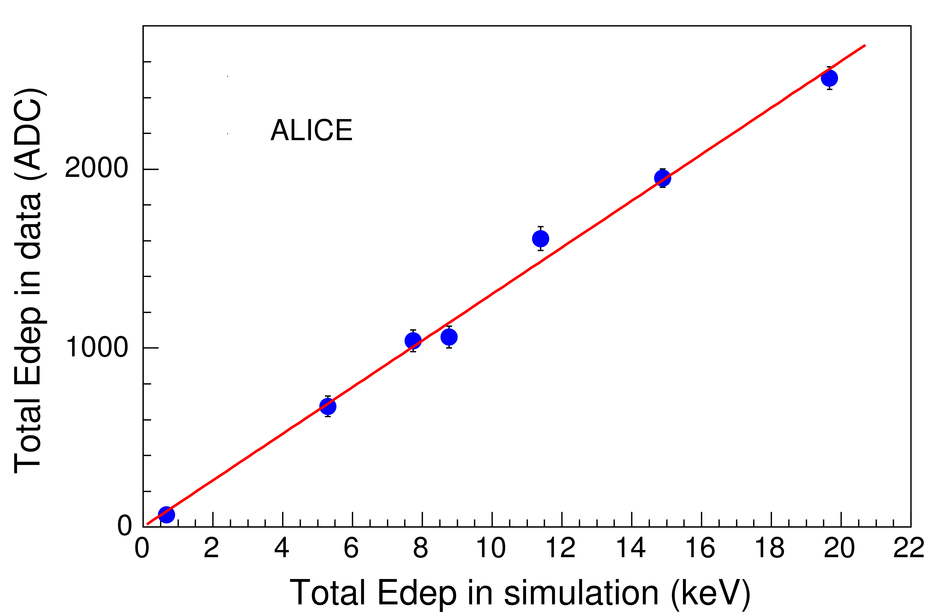## Figure 3

 Probability distribution of themeasured $N_{\rm {\gamma-like}}$ clusters (number of clusters above thephoton-hadron discrimination thresholds)in pp collisions at $\sqrt{s}$ = 0.9, 2.76 and 7 TeV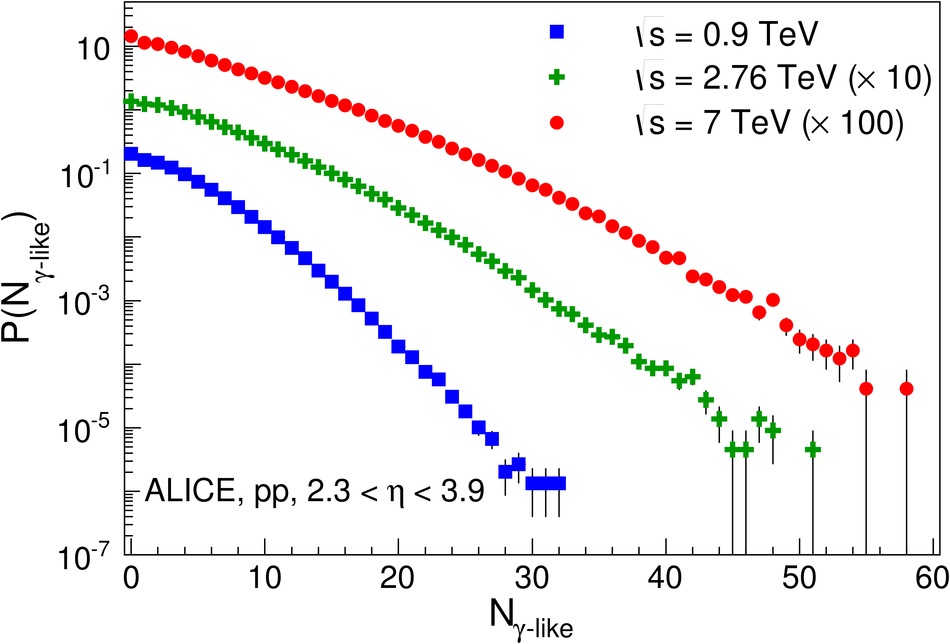## Figure 4

 Detector response matrices inpp collisions at $\sqrt{s}$ = 0.9, 2.76 and 7 TeV using PHOJET event generator. $N_{\rm {\gamma-like}}$ and $N_{\rm{\gamma-true}}$ denote the measured and true photon multiplicities, respectively## Figure 5

 Test of the unfolding method using PHOJET eventgenerator for pp collisions at $\sqrt{s}$ = 0.9 (top), 2.76 (middle)and 7 TeV (bottom). The measured, unfolded and true photon multiplicities are presented The lower panels show the ratios of unfolded to true multiplicity distributions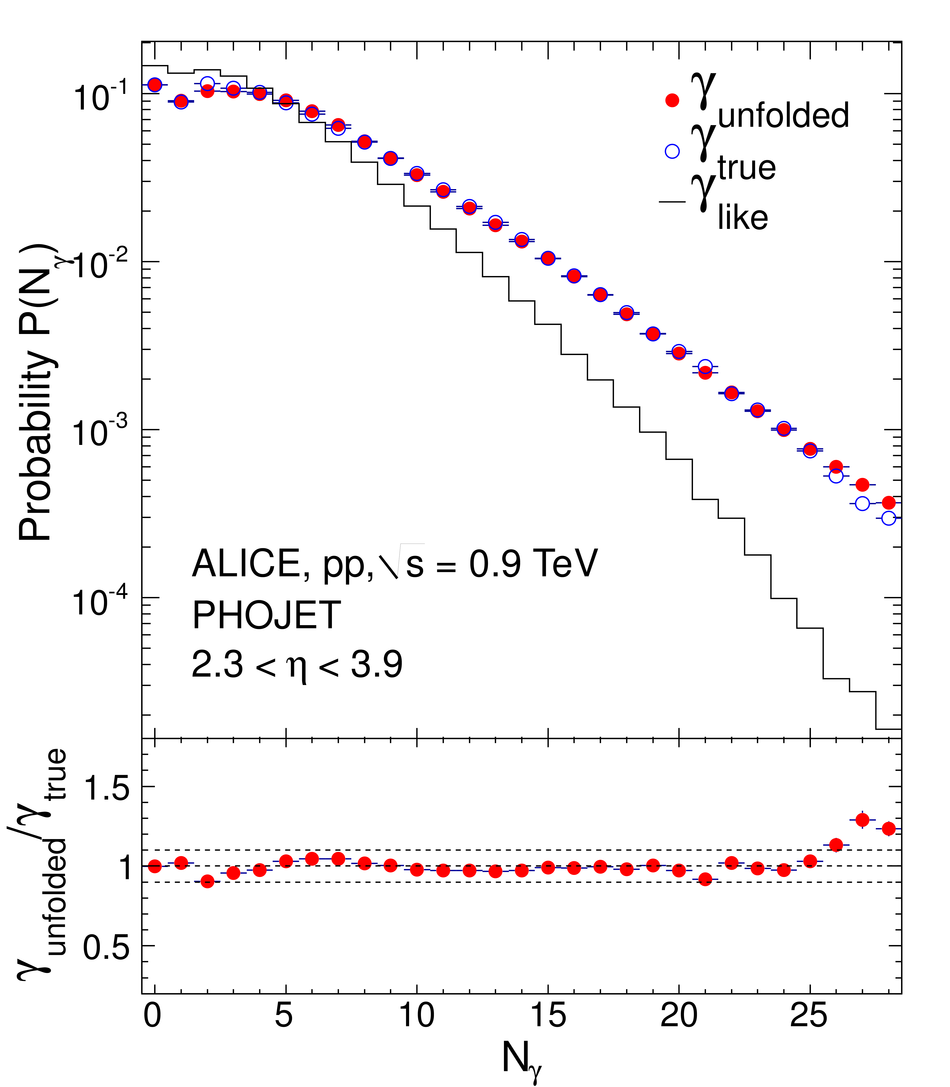## Figure 6

 Multiplicity distributions of photons within $2.3 < \eta < 3.9$ forinelastic eventsin pp collisions at $\sqrt{s}$ = 0.9 (top), 2.76 (middle)and 7 TeV (bottom). Predictions from differentevent generators are superimposed. Lower panelsshow the ratios between the data and MC distributions The error bars are statisticaland the shaded regions represent the systematicuncertainties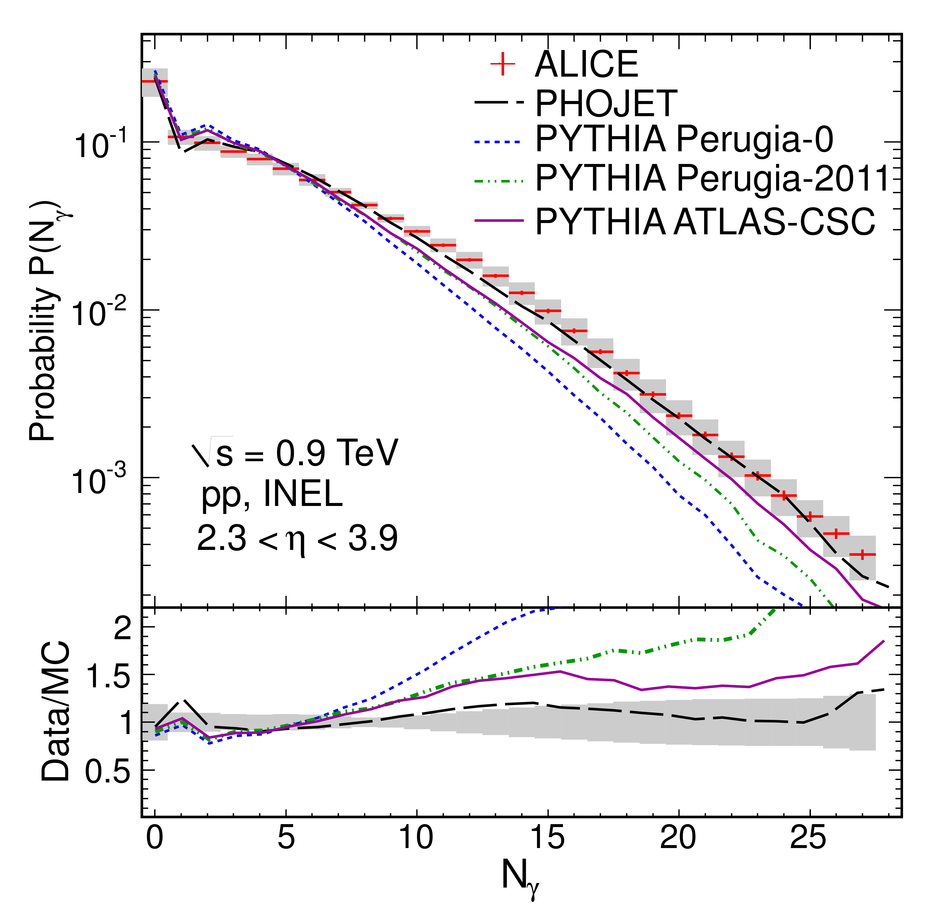## Figure 7

 Multiplicity distribution of photons for inelastic collisions, fitted to single NBD functionsfor pp collisions at $\sqrt{s}$ = 0.9, 2.76 and 7 TeV The error bars are statisticaland the shaded regions represent the systematicuncertainties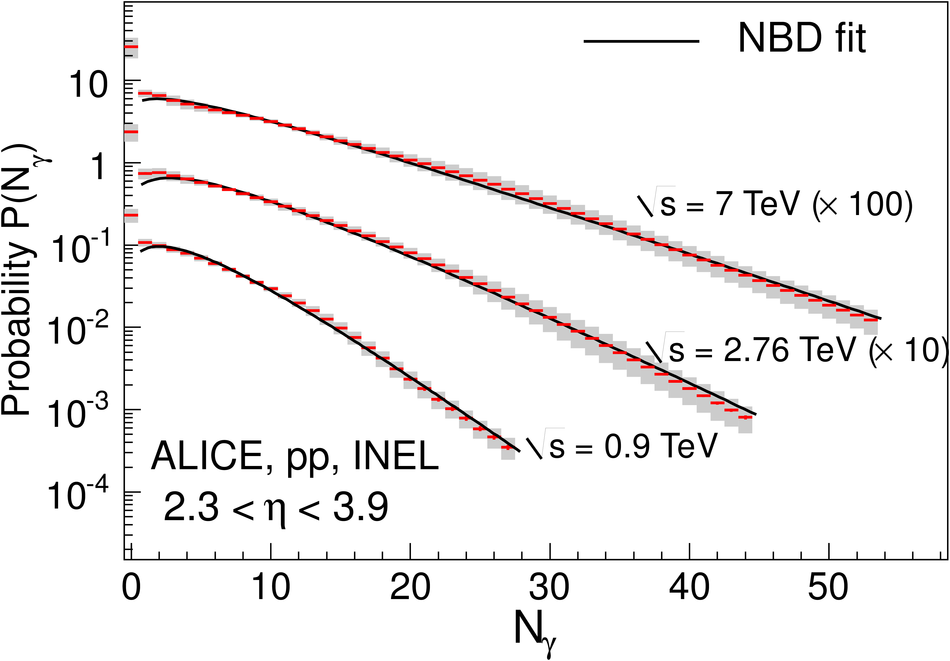## Figure 8

 Upper panel shows the photon multiplicity distributions in terms of KNO variable for ppcollisions at $\sqrt{s}$ = 0.9, 2.76 and 7 TeV for inelastic collisions Lower panel shows the ratios of thedistributions for 2.76 and 7 TeV with respect to 0.9 TeV## Figure 9

 Average photon multiplicity within $2.3 < \eta < 3.9$ as a function of center of mass energy for pp collisions. The datapoints from UA5 experiment are superimposed on the ALICEdata. The energy dependence for the NSD events is consistent with botha logarithmic and a power-law fit## Figure 10

 Pseudorapidity distribution of photons for inelastic eventsfor pp collisions at $\sqrt{s}$ = 0.9 (top), 2.76 (middle)and 7 TeV (bottom). Results from different event generatorsare superimposed. The statistical errors are within the symbol sizes andthe shaded regions represent the systematic uncertainties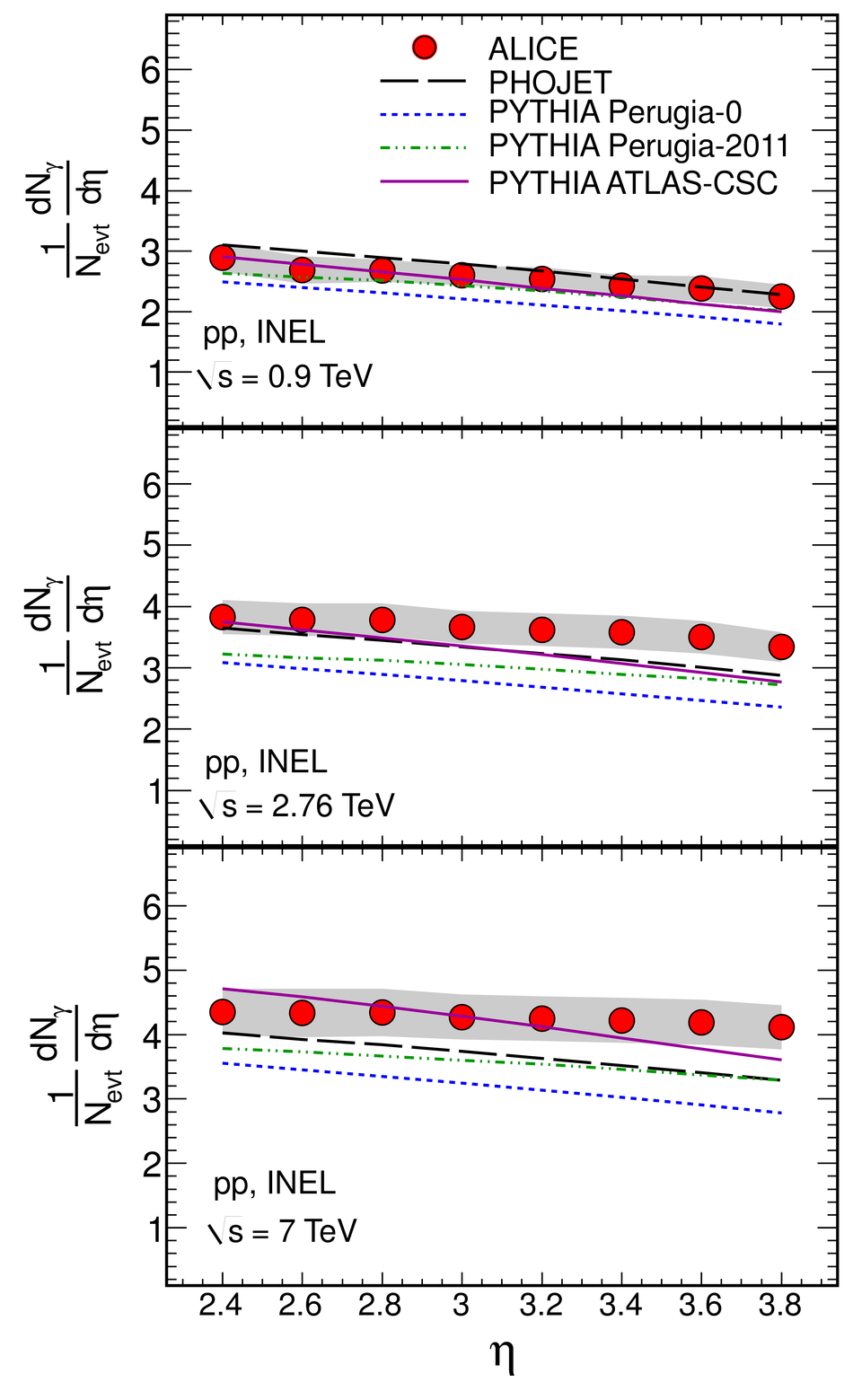## Figure 11

 Pseudorapidity distribution of photons for inelastic events as a function of $\eta - y_{\rm beam}$ for pp collisions at $\sqrt{s}$ = 0.9, 2.76 and 7 TeV. Correspondingdistributions from PYTHIA (tune ATLAS-CSC) are superimposed The statistical uncertainties are within symbol sizes andthe shaded regions represent the systematic uncertainties